Getting Started Guide 7.5

Chapter 9,
Getting Started with Math

LibreOffice’s Formula (Equation) Editor

This document is Copyright © 2023 by the LibreOffice Documentation Team. Contributors are listed below. You may distribute it and/or modify it under the terms of either the GNU General Public License (https://www.gnu.org/licenses/gpl.html), version 3 or later, or the Creative Commons Attribution License (https//creativecommons.org/licenses/by/4.0/), version 4.0 or later.

All trademarks within this guide belong to their legitimate owners.

## Contributors

To this edition

 Peter Schofield Jean H. Weber Olivier Hallot

To previous editions

 Skip Masonsmith Kees Kriek Peter Schofield Kees Kriek Mitchell Camfield Rafael Lima Winston Min Tjong Hazel Russman Jean Hollis Weber John A Smith Martin Saffron Regina Henschel Christian Kühl Florian Reisinger Gisbert Friege (Dmaths) Jochen Schiffers Frédéric Parrenin Bernard Siaud Steve Fanning Olivier Hallot Roman Kuznetsov Dave Barton Laurent Balland-Poirier

## Feedback

Note

Everything you send to a mailing list or forum, including your email address and any other personal information that is written in the message, is publicly archived and cannot be deleted.

## Publication date and software version

Published July 2023. Based on LibreOffice 7.5 Community.
Other versions of LibreOffice may differ in appearance and functionality.

## Using LibreOffice on macOS

Some keystrokes and menu items are different on macOS from those used in Windows and Linux. The table below gives some common substitutions for the instructions in this document. For a detailed list, see the application Help.

 Windows or Linux macOS equivalent Effect Tools > Options menu selection LibreOffice > Preferences Access setup options Right-click Control+click or right-click depending on computer setup Open a context menu Ctrl (Control) ⌘ (Command) Used with other keys Alt ⌥ (Option) or Alt Used with other keys F11 ⌘+T Open the Sidebar Styles deck

# Introduction

Math is a formula editor included with LibreOffice used to create or edit formulas (equations) in a symbolic form, within LibreOffice documents or as stand-alone objects. Example formulas are shown below:

$\frac{\mathit{df}\left(x\right)}{\mathit{dx}}=\mathrm{ln}\left(x\right)+{\mathrm{tan}}^{-1}\left({x}^{2}\right)$ or ${\mathrm{NH}}_{3}+{\mathrm{H}}_{2}\mathrm{O}\mathrm{⇌}{\mathrm{NH}}_{4}^{+}+{\mathrm{OH}}^{\phantom{\rule{0.5em}{0ex}}-}$

The Formula Editor in Math uses a markup language to represent formulas. This markup language is designed to be easily read wherever possible. For example, a over b using markup language produces the fraction $\frac{a}{b}$ when used in a formula.

# Getting started

The Formula Editor creates a formula as a separate file for a formula library or insert formulas directly into a document using LibreOffice Writer, Calc, Impress, or Draw.

## Formulas as separate documents or files

To create a formula as a separate document or file, use one of the following methods to open an empty formula document in LibreOffice Math (Figure 7):

• On the Menu bar, go to File > New > Formula.

• On the Standard toolbar, click the triangle to the right of the New icon and select Formula.

• In the Start Center, click Math Formula.

Figure 1: LibreOffice Math window parts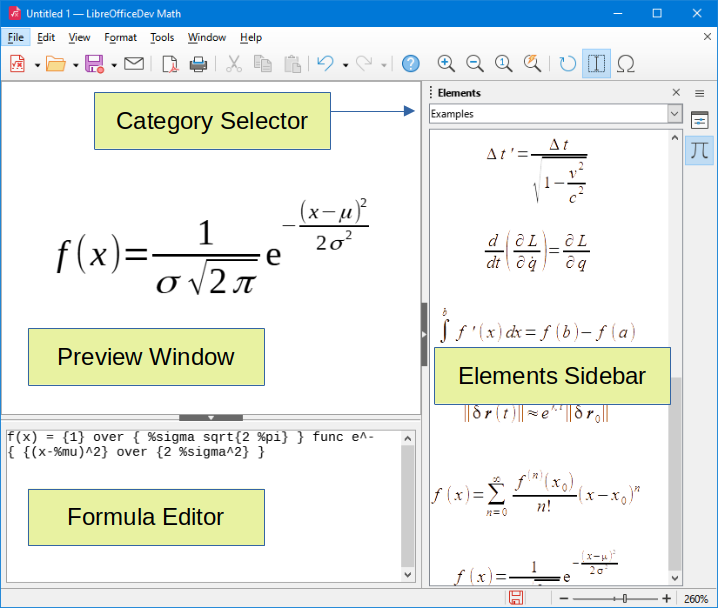• From within LibreOffice Math, use the keyboard shortcut Ctrl+N.

As the markup language in the Formula Editor is entered, the formula will appear in the Preview window during and after input of the markup language. The Elements panel on the sidebar to the right of the Preview window may also appear, if it has been selected in View on the Menu bar (Figure 2).

For more information on creating formulas, see “Creating formulas” on page 1.

Figure 2: Enabling the Elements panel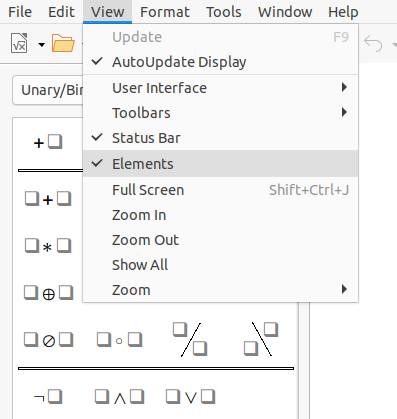## Formulas in LibreOffice documents

To insert a formula into a LibreOffice document, open the document in Writer, Calc, Draw, or Impress. The LibreOffice component in use affects how to position the cursor to insert the formula.

• In Writer, click in the paragraph where the formula should be inserted.

• In Calc, click in the spreadsheet cell where the formula should be inserted.

• In Draw and Impress, the formula is inserted into the center of the drawing or slide.

Then, go to Insert > Object > Formula Object on the Menu bar to open the Formula Editor. Alternatively, go to Insert > Object > OLE Object on the Menu bar to open the Insert OLE Object dialog, then select Create new, choose the Object Type “LibreOffice 7.5 Formula” and then click OK to open the Formula Editor.

The Elements panel to the right of the Preview window if Elements has been selected in View on the Menu bar.

Figure 3 shows an example Writer document with the formula box selected ready for a formula to be entered.

After entering the markup language for the formula, close the Formula Editor by pressing the Esc key or by clicking an area outside the formula in the document. Double-click on the formula object in the document to open the Formula Editor again to edit the formula.

Formulas are inserted as OLE objects into documents and can be placed in different ways. For more information on OLE objects, see “Formulas in Writer” on page 1, “Formulas in Calc, Draw, and Impress” on page 1, and the user guides for Writer, Calc, Draw, and Impress

Figure 3: Empty formula in a Writer document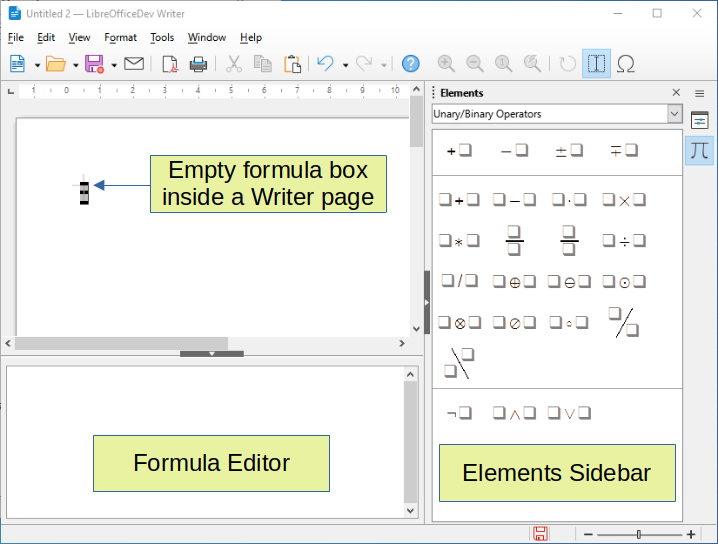.

For frequent formula insertion, it is recommended to add the Formula button to the Standard toolbar or create a keyboard shortcut. See “Customization” on page 1 for more information.

# Creating formulas

Create a formula using one of the following methods:

• In the Elements panel, select a category in the drop-down list, then select a symbol.

• Right-click in the Formula Editor and select a category, then select a symbol in the context menu.

• Enter markup language directly in the Formula Editor.

Note

Using the Elements panel or the context menus to create a formula provides a convenient way to learn the markup language used by Math.

Tip

When using the Elements panel, it is recommended to have Extended tips selected in the LibreOffice Options. This will help to identify the categories and symbols to use in the formula. Go to Tools > Options > LibreOffice > General on the Menu bar and select Extended tips in the Help section.

## Elements panel

The Elements panel (Figure 2) is a visual tool to help create and edit formulas. It organizes mathematical symbols and operators using Categories in a drop-down list. The steps below describe how to enable the Elements panel and navigate its categories and symbols.

1)  Go to View > Elements on the Menu bar to open the Elements panel.

2)  Select the category for the formula from the drop-down list at the top of the Elements panel.

3)  Select the symbol for the formula in the Elements panel. The symbols that are available change according to the selected category.

4)  After choosing one of the symbols in the Elements panel, the Formula Editor will be updated with the Markup notation of the selected symbol.

Note

The Elements panel can either be a floating dialog, as shown in Figure 2, or positioned to the right of the Formula Editor, as shown in Figure 3.

Tip

The Elements panel includes an Examples category which provides example formulas to use as a starting point for a formula or equation.

The Formula Editor also provides a context menu to access categories and symbols when creating a formula. Right-click in the Formula Editor to open the context menu. Select a category and then select the markup example for use in the sub-context menu. An example is shown in Figure 4.

Note

The Elements panel and the context menu contain only the most common commands that are used in formulas. Other symbols and commands not listed in the Elements panel and context menu must be inserted manually using the markup language. For a complete list of commands and symbols available in Math, see Appendix A, Commands Reference, in the Math Guide.

Figure 4: Context menu in Formula Editor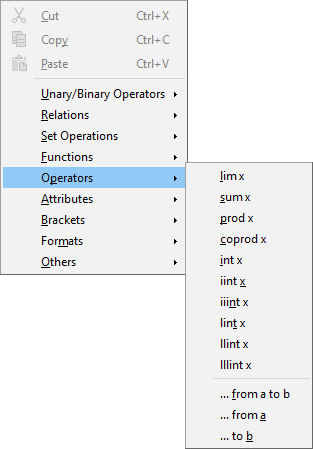## Markup language

Markup language is entered directly into the Formula Editor. For example, typing 5 times 4 into the Formula Editor creates the simple formula $5×4$ . For experienced markup language users, it can be the quickest way to enter a formula. Table 1 shows some examples of using markup language to enter commands. For a full list of commands that can be used in the Formula Editor, see Appendix A, Commands Reference, in the Math Guide.

Table 1: Example commands using markup language

 Display Command Display Command $a=b$ a = b $a$ sqrt {a} $a2$ a^2 $an$ a_n $∫f(x)dx$ int f(x) dx $∑an$ sum a_n $a≤b$ a <= b $∞$ infinity $a×b$ a times b $x⋅y$ x cdot y

## Greek characters

### Using markup language

Greek characters are commonly used in formulas, but they cannot be entered into a formula using the Elements panel or the context menu. Use the English names of Greek characters in markup language when entering Greek characters into a formula. See Appendix A, Commands Reference, in the Math Guide for a list of characters that can be entered using markup language.

• For a lowercase Greek character, type a percentage % sign, then type the character name in lowercase using the English name. For example, typing %lambda creates the Greek character λ.

• For an UPPERCASE Greek character, type a percentage % sign, then type the character name in UPPERCASE using the English name. For example, typing %LAMBDA creates the Greek character Λ.

• For an italic Greek character, type a percentage % sign followed by the i character, then the English name of the Greek character in lower or UPPER case. For example, typing %iTHETA creates the italic Greek character Θ.

### Symbols dialog

Greek characters can also be entered into a formula using the Symbols dialog.

1)  Make sure the cursor is in the correct position in the Formula Editor.

2)  Go to Tools > Symbols on the Menu bar, or click the Symbols icon in the Tools toolbar, to open the Symbols dialog (Figure 5).

3)  Select Greek in the Symbol set drop-down list. For italic characters, select iGreek in the drop-down list.

4)  Double-click the desired Greek character to insert it or select it and click Insert. When selected, the name of the character is shown below the symbol list.

5)  Click Close after entering Greek characters into the formula.

Figure 5: Symbols dialog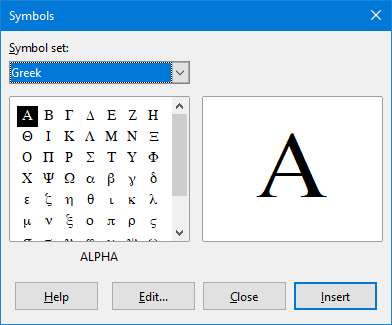## Formula examples

Example 1

The simple formula $5×4$ can be created using LibreOffice Math as follows:

1)  Make sure the cursor is flashing in the Formula Editor, then select the category Unary/Binary Operators and symbol Multiplication using one of the following methods:

• Right-click in the Formula Editor and select Unary/Binary Operators > a times b in the context menu.

• In the Elements panel, select Unary/Binary Operators in the drop-down list and then select the Multiplication icon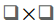.

• Using markup language, enter 5 times 4 in the Formula Editor.

2)  Select the first placeholder <?> before the word times in the Formula Editor and replace it with the character 5. The formula in the document updates automatically.

The first two methods place the formula text <?> times <?> in the Formula Editor and the symbolappears in the document. For the third method, using markup language in the Formula Editor places the formula $5×4$ directly into the document and there is no need to carry out the following steps.

3)  Select the second placeholder <?> after the word times in the Formula Editor and replace it with the character 4. The formula in the document updates automatically.

Tip

To move forward from one placeholder to the next placeholder in a formula, press the F4 key. To move backward from one placeholder to the previous placeholder in a formula, use the key combination Shift+F4.

Note

If necessary, to prevent a formula in a document from updating automatically. Go to View on the Menu bar and deselect AutoUpdate display. To then manually update a formula, press the F9 key or select View > Update on the Menu bar.

Example 2

To create the formula $\mathrm{\pi }\phantom{\rule{2em}{0ex}}\simeq \phantom{\rule{2em}{0ex}}3.14159$ where the value of pi is rounded to 5 decimal places, without knowing markup associated with the Is Similar Or Equal symbol $\simeq$ , follow these steps:

1)  Make sure the cursor is flashing in the Formula Editor.

2)  Enter %pi in the Formula Editor to enter the Greek character for pi (π).

3)  Select the category Relations and symbol Is Similar Or Equal using one of the following methods:

• Right-click in the Formula Editor and select Relations > a simeq b in the context menu.

• In the Elements panel, select Relations in the drop-down list and then select the Is Similar Or Equal icon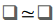.

4)  Delete the first placeholder <?> before the word simeq in the Formula Editor.

5)  Select the second placeholder <?> after the word simeq in the Formula Editor and replace it with the characters 3.14159. The formula $\mathrm{\pi }\phantom{\rule{2em}{0ex}}\simeq \phantom{\rule{2em}{0ex}}3.14159$ now appears in the document.

# Editing formulas

To edit a formula and switch into formula editing mode depends on whether the formula is in Math or another LibreOffice component.

1)  In Math, double-click on a formula element in the formula that appears in the Preview window to select the formula element in the Formula Editor, or directly select a formula element in the Formula Editor.

1. In Writer, Calc, Impress, or Draw, double-click on the formula, or right-click on the formula and select Edit in the context menu, to open the Formula Editor and enter editing mode. The cursor is positioned at the start of the formula in the Formula Editor.

Note

If a formula element cannot be selected using the cursor, click on the Formula Cursor icon in the Tools toolbar to activate the formula cursor.

2)  Select the formula element to be changed using one of the following methods:

• Click on the formula element in the Preview window, positioning the cursor at the beginning of the formula element in the Formula Editor, then select the formula element in the Formula Editor.

• Double-click on the formula element in the Preview window to select the formula element in the Formula Editor.

• Position the cursor in the Formula Editor at the formula element to be edited, then select that formula element.

• Double-click directly on the formula element in the Formula Editor to select it.

3)  Make changes to the selected formula element.

4)  Go to View > Update on the Menu bar, or press the F9 key, or click on the Update icon on the Tools toolbar to update the formula in the Preview window or in the document.

5)  In Math, save changes to the formula after editing.

1. In Writer, Calc, Impress, or Draw, click anywhere in the document away from the formula to leave editing mode, then save the document to save changes to the formula.

# Formula layout

This section provides some advice on how to lay out complex formulas in Math or in a LibreOffice document.

## Using braces

LibreOffice Math knows nothing about order of operations within a formula. Braces (curly brackets) are used to define the order of operations. The following examples show how braces can be used in a formula.

Example 1

2 over x + 1 gives the result $\frac{2}{x}+1$

Math has recognized that the 2 before and the x after the over command as belonging to the fraction, and has represented them accordingly. For $x+1$ rather than $x$ to be the denominator, they must be bracketed together using braces so that both will be placed there.

Inserting braces into 2 over {x + 1} gives the result $\frac{2}{x+1}$ where $x+1$ is now the denominator.

Example 2

– 1 over 2 gives the result $\frac{-1}{2}$

Math has recognized the minus sign as a prefix for the 1 and has therefore placed it in the numerator of the fraction. To show that the whole fraction is negative, with the minus sign in front of the fraction, put the fraction in braces to signify to Math that the characters belong together.

Adding braces into the markup language – {1 over 2} gives the result $–\frac{1}{2}$ and the whole fraction is now negative.

Example 3

When braces are used in markup language, they define the layout of the formula and are not displayed or printed. To use braces within a formula, use the commands lbrace and rbrace within the markup language.

x over {–x + 1} gives the result $\frac{x}{–x+1}$

Replace the braces using the commands lbrace and rbrace in the markup language. Write x over lbrace –x + 1 rbrace and the result is $\frac{x}{\left\{–x+1\right\}}$

## Brackets (parentheses) and matrices

To use a matrix in a formula, the matrix command must be used. Below is a simple example of a 2 x 2 matrix.

 matrix { a # b ## c # d } $abcd$

In matrices, rows are separated by two hashes (##) and entries within each row are separated by one hash (#).

Normally, when brackets are within a matrix, the brackets do not scale as the matrix increases in size. The example below shows a formula where the parentheses do not scale to the size of the resulting matrix.

 ( matrix { a # b ## c # d } ) $(abcd)$

To overcome this problem, Math provides scalable brackets that grow in size to match the size of the matrix. Use the commands left( and right) to create scalable brackets around a matrix. The following example shows how to create a matrix with scalable parentheses.

 left( matrix { a # b ## c # d } right) $(abcd)$

Scalable brackets can also be used with any element of a formula, such as fraction, square root, and so on.

To create a matrix where some values are empty, use the grave accent () so that Math will put a small space in that position, as shown in the example below.

 left( matrix { 1 # 2 # 3 ## 4 #  # 6 } right) $(12346)$

Tip

Use the commands left[ and right] to obtain square brackets. A list of all brackets available within Math can be found in Appendix A, Commands Reference, of the Math Guide.

Tip

To make all brackets to be scalable, go to Format > Spacing on the Menu bar to open the Spacing dialog. Click on Category, select Brackets in the drop-down list, and then select the option Scale all brackets.

## Unpaired brackets

When using brackets in a formula, Math expects that for every opening bracket there will be a closing one. If there is no closing bracket, Math places an inverted question mark next to where the closing bracket should have been placed. For example, lbrace a; b will result in  because the right bracket rbrace is missing.

This inverted question mark disappears when all the brackets are paired. The previous example could be fixed to lbrace a; b rbrace, resulting in $\left\{a;b\right\}$ . However, there are cases where an unpaired bracket is necessary and for that there are a couple of options.

### Non scalable brackets

A backslash \ is placed before a non scalable bracket to indicate that the subsequent character should not be regarded as a bracket, but rather as a literal character.

For example, the unpaired brackets in the formula [ a; b [ would result in an inverted question mark because Math expects that [ will be closed by ]. To fix the error, use the backslash and insert \ [ a; b \ [ into the Formula Editor to obtain $\left[a;b\left[$ as the result.

### Scalable brackets

To create unpaired scalable brackets or braces in a formula, the markup commands left, right, and none can be used.

Example

The following formula uses the stack command to create a two-line formula and adds a bracket only to the right side of the stack.

 abs x = left lbrace stack {x "for" x >= 0 # -x "for" x < 0} right none $|x|={xforx≥0−xforx<0$

This effect can be achieved by using the left lbrace command combined with the right none command. The first command indicates that the left bracket is a lbrace whereas the second command tells Math that the right bracket will be none, meaning that no bracket will be added to the right side of the formula.

## Recognizing functions

In the basic installation of Math, Math outputs functions in normal characters and variables in italic characters. However, if Math fails to recognize a function, the markup command func before a function can force Math to recognize any following text as a function and uses normal characters.

For a full list of functions within Math, see Appendix A, Commands Reference, in the Math Guide.

Some Math functions have to be followed by a number or a variable. If these are missing, Math places an inverted question mark where the missing number or variable should be. To remove the inverted question mark and correct the formula, enter a number, a variable, or a pair of empty brackets as a placeholder.

Tip

Use the F3 key to navigate to the next formula error or the key combination Shift+F3 to move to the previous error.

## Formulas over multiple lines

To create a formula that requires more than one line, do not press the Enter key to create a new line -- the markup language in the Formula Editor goes to a new line, but the resulting formula does not have two lines. To add a new line to the formula, use the markup command newline.

Example

The examples below illustrate how the markup command newline can be used to add a new line to a formula. The first one simply adds an Enter to break the line in the markup language, which does not result in adding a new line. The second example uses the newline command, yielding a two-line formula.

 Markup Language Resulting Formula x = 3y = 1 $x=3y=1$ x = 3 newline y = 1 $x=3y=1$

It is not possible in Math to create multiple line formulas when a line ends with an equals sign and to continue the formula on a new line without completing the term on the right side of the equals sign. If a multiple line formula is required to have an equals sign at the end of a line without a term after the equals sign, then use either empty quotes “” or empty braces {} or the space characters grave  or tilde ˜.

By default, a multiple line formula is center aligned. For more information on alignment using the equals sign, see “Customization” on page 1.

## Spacing within formulas

Spacing between the elements in a formula is not set by using space characters in the markup language. To add spaces into a formula, use one of the following options:

• Grave  to add a small space.

• Tilde ~ for a large space.

• Add space characters between quotes “ ”. These spaces will be considered as text.

Any spaces at the end of a line in the markup language are ignored by default.

## Adding limits to sum/integral commands

The sum and int commands, used for summations and integrals respectively, can take the parameters, from and to, to set the lower and upper limits. The parameters from and to can be used singly or together as shown by the following examples. For more information on the sum and integral commands, see Appendix A, Commands Reference, in the Math Guide.

Examples

Here are some examples of how to add upper and lower bounds to summations and integrals. Note that brackets can be used to explicitly define which parts of the formula correspond to the desired bounds.

 Markup Language Resulting Formula sum from k = 1 to n a_k $∑k=1nak$ sum to infinity 2^{-n} $∑∞2−n$ sum from{ i=1 } to{ n } sum from{ j=1; i <> j } to{ m } x_ij $∑i=1n∑j=1;i≠jmxij$ int from 0 to x f(t) dt $∫0xf(t)dt$ int_0^x f(t) dt $∫0xf(t)dt$ int from Re f $∫ℜf$

## Writing derivatives

When writing derivatives, use the over command to identify fractions to Math. The over command is combined with the character d for a total derivative or the partial command for a partial derivative to achieve the effect of a derivative. Braces {} are used in each side of the elements to surround them and make the derivative, as shown by the following examples.

Examples

Below are a few examples of using Math to write derivatives.

 Markup Language Resulting Formula {df} over {dx} $dfdx$ {partial f} over {partial y} $∂f∂y$ {partial^2 f} over {partial t^2} $∂2f∂t2$

Note

To write function names with primes, as is normal in school notation, first add the symbols to the catalog. See “Catalog customization” on page 1 for more information.

## Markup language characters as normal characters

Characters that are used as controls in markup language cannot be entered directly as normal characters. These characters are: %, {, }, &, |, _, ^ and ". For example, 2% = 0.02 in markup language will not appear in the formula with the same characters. To overcome this limitation in markup language, use one of the following methods:

• Use double quotes to mark that character as text, for example 2"%"= 0.02 will appear in a formula as $2\text{%}=0.02$ . However, this method cannot be used for the double-quote character itself, see “Text in formulas” below.

• Add the character to the Math Catalog, for example the double quote character.

• Use commands, for example lbrace and rbrace provide literal braces $\left\{\right\}$ .

Note

The Special Characters dialog used by other LibreOffice components is not available in Math. For regular use of special characters in Math, adding the characters to the Math Catalog is recommended; see “Catalog customization” on page 1 for more information.

## Text in formulas

To include text in a formula, enclose any text in double-quotes, for example x " for " x >= 0 in markup language will create the formula $x\text{for}x\ge 0$ . All characters, except double quotes, can be used in text.

However, for double quotes in formula text, create the text with double quotes in LibreOffice Writer, then copy and paste the text into the Formula Editor, as shown in Figure 6.

Figure 6: Example of double quotes in formula text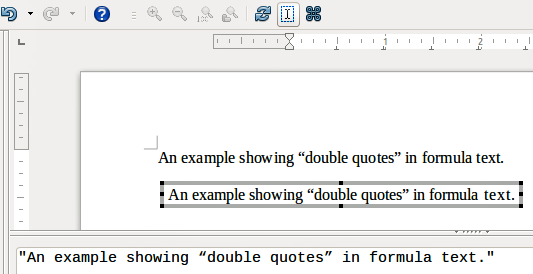The font used for text in a formula will be the default font that has been set in the Fonts dialog. For more information on how to change fonts used for in formulas, see “Changing formula appearance” on page 1.

By default, text alignment is left-justified in formulas. For more information on how to change text alignment, see “Adjusting formula alignment” on page 1.

## Formatting text in formulas

Formatting commands are not interpreted within text used in formulas. To use formatting commands within formula text, break up the text using double quotes in the Formula Editor.

Example

Enter the following in the Formula Editor:

"In " color blue bold "isosceles" "triangles, the base angles are equal"

creates the following text in a formula $\text{In}\text{isosceles}\text{triangles, the base angles are equal}$ .

This example shows how to use the markup commands color and bold to format the word “isosceles”. Note that the commands are applied to the immediately subsequent text within double quotes. Hence, the formatting commands do not apply to the remainder of the text in the example because it is in a separate quote block.

The command color needs to be followed by the name of the desired color or to its RGB or hexadecimal value. Refer to Appendix A, Command Reference, in the Math Guide for a list of predefined color names.

## Aligning formulas using equals sign

LibreOffice Math does not have a command for aligning formulas on a particular character. However, a matrix can align formulas on a character and this character is normally the equals sign (=). In addition, the markup commands alignr, alignl and alignc can set the alignment of each value inside the matrix to the right, left or center, respectively.

Example

The example below uses a matrix to align formulas on the equals sign. Notice how the alignment commands align the contents of each position in the matrix.

 matrix{ alignr x+y # {}={} # alignl 2 ## alignr x # {}={} # alignl 2-y } $x+y=2x=2−y$

Note

The empty braces each side of the equals sign are necessary because the equals sign is a binary operator and requires an expression on each side. Spaces, or  or ~ characters each side of the equals sign can be used, but braces are recommended as they are easier to see within the markup language.

The spacing on each side of the equals sign can be reduced by changing the inter-column spacing of the matrix. See “Adjusting formula spacing” on page 1 for more information.

# Changing formula appearance

This section describes how to change the font or font size in a selected formula and how to change the default font or font size.

Note

Only formulas inserted after the change in font settings will use the new default settings. The font settings of formulas previously inserted would have to be updated individually to match the same font settings as the new default settings.

Tip

The extension “Formatting of all Math formulas” allows the changing of font name and font size for all or only for selected formulas in a document. The download and the installation and usage instructions are here: https://extensions.libreoffice.org/en/extensions/show/formatting-of-all-math-formulas

## The Properties panel in the sidebar

This very simple panel contains buttons to set fonts, fonts sizes, spacing and alignment of formulas (Figure 7).

Figure 7: Properties panel on the sidebar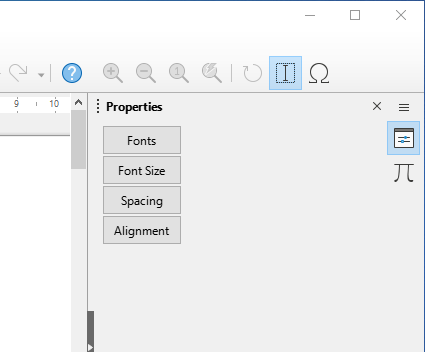## Formula font size

### Current formula font size

To change the font size used for a formula already inserted in Math or another LibreOffice component:

1)  Click in the markup language in the Formula Editor.

2)  Go to Format > Font size on the Menu bar or click on the Font Size button on the sidebar to open the Font Sizes dialog (Figure 8).

3)  Select a different font size using the Base size spinner or type a new font size in the Base Size box.

4)  Click OK to save changes and close the dialog. An example result when the font size is changed is shown below.

Example

Default font size 12pt: $\mathrm{\pi }\phantom{\rule{2em}{0ex}}\simeq \phantom{\rule{2em}{0ex}}3.14159$

After font size change to 18pt: $\mathrm{\pi }\phantom{\rule{2em}{0ex}}\simeq \phantom{\rule{2em}{0ex}}3.14159$

Figure 8: Font Sizes dialog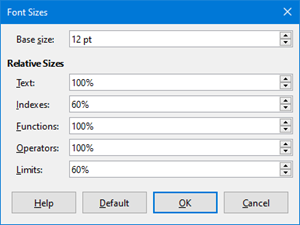### Default formula font size

To change the default font size used for all formulas in Math or another LibreOffice component:

1)  Before inserting any formulas into a document, go to Format > Font size on the Menu bar or click on the Font Size button on the sidebar to open the Font Sizes dialog (Figure 8).

2)  Select a different font size using the Base size spinner or type a new font size in the Base size box.

3)  Click Default and confirm the changes to the base size font. Any formulas created from this point on will use the new base size font for formulas.

4)  Click OK to save changes and close the Font Sizes dialog.

### Font size options

The Font Sizes dialog (Figure 8) specifies the font sizes for a formula. Select a base size and all elements of the formula will be scaled in relation to this base.

• Base size – all elements of a formula are proportionally scaled to the base size. To change the base size, select or type in the desired point (pt) size. Other units of measure or other metrics can also be automatically converted to points. For example, entering 1in or 1”, Math converts the value to 72 pt.

• Relative Sizes – in this section, determine the relative sizes for each type of element with reference to the base size.

• Default – click this button to save any changes as a default for all new formulas. A confirmation message appears.

## Formula fonts

### Current formula fonts

To change the fonts used for the current formula in Math or another LibreOffice component:

1)  Click in the markup language in the Formula Editor.

2)  Go to Format > Fonts on the Menu bar or click on the Fonts button on the sidebar to open the Fonts dialog (Figure 9).

Figure 9: Fonts dialog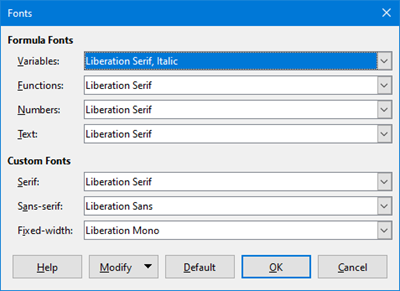3)  Select a new font for each of the various options in the drop-down lists.

4)  If the desired font is not in the drop-down list, click Modify and select the option in the context menu to open a fonts dialog. Select the desired font and click OK to add it to the drop-down list for that option.

5)  Click OK to save changes and close the Fonts dialog.

### Default formula fonts

To change the default fonts used for all formulas in Math or another LibreOffice component:

1)  Before inserting any formulas in a document, go to Format > Fonts on the Menu bar or click on the Fonts button on the sidebar to open the Fonts dialog (Figure 9).

2)  Select a new font for each of the various options in the drop-down lists.

3)  If the desired font is not in the drop-down list, click Modify and select the option in the context menu to open a fonts dialog. Select a font, and click OK to add it to the drop-down list for that option.

4)  Click Default and confirm changes to the fonts. Any formulas created from this point on will use the new font for formulas.

5)  Click OK to save changes and close the Fonts dialog.

### Formula font options

Defines the fonts that can be applied to formula elements.

• Formula Fonts – defines the fonts used for the variables, functions, numbers and inserted text that form the elements of a formula.

• Custom Fonts – in this section of the Fonts dialog (Figure 9), fonts are defined which format other text components in a formula. The three basic fonts Serif, Sans, and Fixed are available. Other fonts can be added to each standard installed basic font using the Modify button. Every font installed on a computer system is available for use.

• Modify – click one of the options in the drop-down menu to access the Fonts dialog, where the font and attributes can be defined for the respective formula and for custom fonts.

• Default – click this button to save any changes as a default for all new formulas. A confirmation message appears.

Note

When a new font is selected for a formula, the old font remains in the list alongside the new one and can be selected again.

Note

Variables should be written in italics, so make sure that the Italic option is selected for the desired font. For all other elements, use the basic form of a font. The style can be easily altered in the formula itself by using the commands italic or bold to set these characteristics and nitalic or nbold to unset them.

Use the Spacing dialog (Figure 10) to determine the spacing between formula elements. The spacing is specified as a percentage in relation to the defined base size for font sizes.

Figure 10: Spacing dialog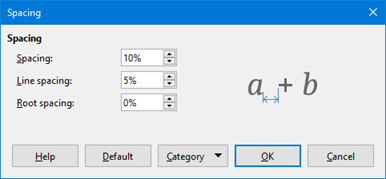### Current formula spacing

To change the spacing used for the current formula in Math or another LibreOffice component:

1)  Click in the markup language in the Formula Editor.

2)  Go to Format > Spacing on the Menu bar or click on the Spacing button on the sidebar to open the Spacing dialog (Figure 10).

3)  Click Category and select one of the options in the drop-down list. The options in the Spacing dialog change according to the category selected.

4)  Enter new values for the spacing category and click OK.

5)  Check the result in the formula.

### Default formula spacing

To change the default spacing used for all formulas in Math or another LibreOffice component:

1)  Before inserting any formulas into a document, go to Format > Spacing on the Menu bar or click on the Spacing button on the sidebar to open the Spacing dialog (Figure 10).

2)  Click Category and select one of the options in the drop-down list. The options in the Spacing dialog change according to the category selected.

3)  Enter new values for the spacing category.

4)  Click Default and confirm changes to the formula spacing. Any formulas created from this point on will use the new spacing for formulas.

5)  Click OK to save changes and close the Spacing dialog.

Note

Only formulas inserted after the change in default spacing will use the new default settings. The spacing of formulas previously inserted would have to be updated individually to match the same spacing as the new default settings.

### Spacing options

Use Category in the Spacing dialog (Figure 10) to determine the formula element to be updated. The appearance of the dialog depends on the selected category. A preview window shows which spacing is modified through the respective boxes.

• Category – defines the selection for the spacing change.

• Spacing – defines the spacing between variables and operators, between lines, and between root signs and radicals.

• Indexes – defines the spacing for superscript and subscript indexes.

• Fractions – defines the spacing between the fraction bar and the numerator or denominator.

• Fraction Bars – defines the excess length and line weight of the fraction bar.

• Limits – defines the spacing between the sum symbol and the limit conditions.

• Brackets – defines the spacing between brackets and the content.

• Excess size (left/right) – determines the vertical distance between the upper edge of the contents and the upper end of the brackets.

• Spacing – determines the horizontal distance between the contents and the upper end of the brackets.

• Scale all brackets – scales all types of brackets. By entering (a over b) in the Formula Editor, the brackets will surround the whole height of the argument. Normally, this is done by entering left (a over b right).

• Excess size – adjusts the percentage excess size. At 0% the brackets are set so that they surround the argument at the same height. The higher the entered value is, the larger the vertical gap between the contents of the brackets and the external border of the brackets. The field can only be used in combination with Scale all brackets.

• Matrices – defines the relative spacing for the elements in a matrix.

• Line spacing – determines the spacing between matrix elements in a row.

• Column spacing – determines the spacing between matrix elements in a column.

• Symbols – defines the spacing of symbols in relation to variables

• Primary height – defines the height of the symbols in relation to the baseline.

• Minimum spacing – determines the minimum distance between a symbol and variable.

• Operators – defines the spacing between operators and variables or numbers.

• Excess size – determines the height from the variable to the operator upper edge.

• Spacing – determines the horizontal distance between operators and variables.

• Borders – adds a border to a formula. This option is particularly useful for integrating the formula into a text file in Writer. When making settings, be sure to not use 0 as a size as this creates viewing problems for text that surrounds the insertion point.

• Preview Field – displays a preview of the current selection.

• Default – saves any changes as default settings for all new formulas. A confirmation dialog will appear before saving these changes.

The alignment settings determine how formula elements located above one another are aligned horizontally relative to each other.

Note

It is not possible to align formulas on a particular character and formula alignment does not apply to text elements. Text elements are always aligned left.

Note

Independent of using formula alignment given below, it is possible to align formulas using the commands alignl, alignc and alignr. These commands also work for text elements.

### Current formula alignment

To change the alignment used for the current formula in Math or another LibreOffice component:

1)  Click in the markup language in the Formula Editor.

2)  Go to Format > Alignment on the Menu bar or click on the Alignment button on the sidebar to open the Alignment dialog (Figure 11).

3)  Select either Left, Centered, or Right for horizontal alignment.

4)  Click OK and check the result in the formula.

Figure 11: Alignment dialog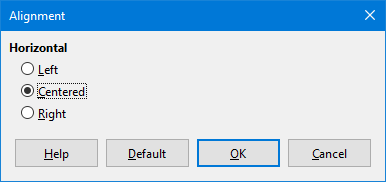Note

Regardless of the alignment option selected in the Alignment dialog, it is possible to align sections of a formula using the commands alignl, alignc and alignr. For example, they can be useful to align formulas in matrices. These commands also work for text elements.

### Default formula alignment

To change the default alignment used for all formulas in Math or another LibreOffice component:

1)  Before inserting any formulas into a document, go to Format > Alignment on the Menu bar or click on the Alignment button on the sidebar to open the Alignment dialog (Figure 11).

2)  Select either Left, Centered, or Right for horizontal alignment.

3)  Click Default and confirm changes to the formula alignment. Any formulas created from this point on will use the new alignment for formulas.

4)  Click OK and check the result in the formula.

Note

Only formulas inserted after the change in default alignment will use the new default settings. The alignment of formulas previously inserted would have to be updated individually to match the same alignment as the new default settings.

## Changing formula color

The color of characters used in a formula can be changed by using the markup language command color followed by a color name, RGB value, or HTML hex value.

The color command only works on the formula element immediately after the color name, RGB value, or hex value. For example, entering the markup language color red 5 times 4 gives the result $5×4$ . Note that only the number 5 was colored red.

To change the color of the whole formula, enclose the whole formula within brackets. For example, entering the markup language color red {5 times 4} gives the result $5×4$ .

### Named colors

For information on the named colors available in Math, see Appendix A, Commands Reference, in the Math Guide. These colors are listed in the Attributes section of the Elements panel (Figure 2 above).

### RGB values

To use custom colors defined by RGB (Red, Green and Blue) values ranging from 0 to 255, use the color rgb R G B markup command, where R, G, and B correspond to the Red, Green, and Blue values of the desired color.

Example

In this example, the term “decision variable” uses the color defined by the RGB values 160, 82, 45.

"Let " x_ij " be a " color rgb 160 82 45 "decision variable " "in the problem under consideration"

Math will create the following output:

$Let xij be a decision variable in the problem under consideration$

### Hex values

Math now supports HTML colors defined by a hex number. Use the color hex 000000 markup command, where 00000 is the corresponding hex number. For example, the command color hex FF0000 decision creates the output $\mathit{decision}$ .

## Background color

It is not possible to select a background color for formulas in LibreOffice Math. The background color for a formula is by default the same color as the document or frame that the formula has been inserted into. In LibreOffice Writer, use object properties to change the background color for a formula. For more information, see “Background and borders” on page 1.

# Formula library

For reuse of formulas, a formula library can be created of the formulas created by the Formula Editor. Individual formulas can be saved as separate files using the ODF format for formulas (file type .odf), or in MathML format (file type .mml).

LibreOffice Math, Writer, Calc, Draw, or Impress can create formulas and build up a formula library.

## Using Math

1)  Create a file folder to contain the formula library.

2)  In LibreOffice, go to File > New > Formula on the Menu bar, or click on Math Formula in the Start Center to open Math and create a formula using the Formula Editor. See “Formulas as separate documents or files” on page 1 for more information.

3)  Go to File > Save As on the Menu bar or use the keyboard shortcut Ctrl+Shift+S to open a Save As dialog.

4)  Navigate to the formula library folder created in step 1.

5)  Name the formula in the File name box.

6)  Select in the File type drop-down list either ODF Formula (.odf) or MathML 2.0 (.mml) as the file type for the formula.

7)  Click Save to save the formula and close the Save As dialog.

## Using Writer, Calc, Draw, or Impress

1)  Create a file folder to contain the formula library.

2)  Open a document using Writer, Calc, Draw, or Impress.

3)  Go to Insert > Object > Formula Object on the Menu bar to open the Formula Editor and create a formula. See “Formulas in LibreOffice documents” on page 1 for more information.

4)  Right-click on the formula object and select Save Copy as in the context menu to open a Save As dialog.

5)  Navigate to the folder created for the formula library.

6)  Name the formula in the File name box.

7)  Select in the File type drop-down list either ODF Formula (.odf) or MathML 2.0 (.mml) as the file type for the formula.

8)  Click Save to save the formula and close the Save As dialog.

## Using a formula library

A formula from the formula library can neither be added to a document by dragging and dropping using the mouse, nor by using Insert > File on the Menu bar. The formula must be inserted as an OLE object.

1)  Open the document in Writer, Calc, Draw, or Impress.

2)  Go to Insert > Object > OLE Object on the Menu bar to open the Insert OLE Object dialog.

3)  Select the option Create from file.

4)  Click Search to open a file browser dialog.

5)  Navigate to the formula library folder.

6)  Select the formula to be inserted and click Open, or double-click on the formula.

7)  Click OK to insert the formula as an OLE object in the document and close the dialog.

# Formulas in Writer

When a formula is inserted into a document, LibreOffice Writer places the formula in a frame and treats the formula as an OLE object. Double-click on an inserted formula to open the Formula Editor in LibreOffice Math, to edit it.

This section explains the options available to change each individual formula within a Writer document. Please refer to the chapters on styles in the Writer Guide for information on how to change the default settings for frame styles for OLE objects.

## Automatic formula numbering

Automatic numbering of formulas for cross-reference purposes can only be carried out in LibreOffice Writer. The easiest way to add numbered formulas in sequence is to use the AutoText entry fn (for 'formula numbered').

1)  Start a new line in the document.

2)  Type fn and then press the F3 key. A two column table with no borders is inserted into the document with the left column containing a sample formula and the right column containing a reference number, as shown below.

 $E=mc2$ (1)

3)  Delete the sample formula and insert the formula as an object in the left column.

4)  Alternatively, first insert the formula into the document, then carry out Steps 1 and 2 above replacing the sample formula with another formula.

## Cross-referencing

1)  Click in the document where the cross-reference should appear.

2)  Go to Insert > Cross-reference on the Menu bar to open the Fields dialog (Figure 12).

3)  Click on the Cross-references tab, then select Text in the Type section.

4)  In the Selection section, select the formula number to refer to.

5)  In the Insert reference to section, select Reference and click Insert.

6)  After creating the cross-references, click Close to close the Fields dialog.

Figure 12: Fields dialog – Cross-references tab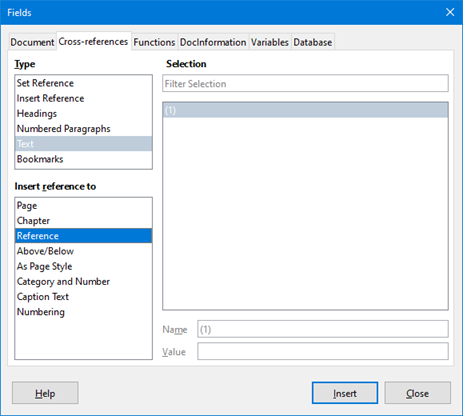Tip

To insert the cross-reference number without parentheses, select Numbering instead of Reference in the Insert reference to section.

Note

To use square parentheses instead of round ones, or to have the cross-reference number be separated from the formula by tabs instead of using a table, then refer to the Writer Guide.

## Anchoring formulas

A formula is treated as an object within Writer and its default anchoring is To character within a paragraph when it is inserted into a document. To change the anchoring of a formula object:

1)  Right-click on the selected formula object and select Anchor in the context menu.

2)  Select a new anchoring option in the context sub-menu. The anchoring positions available are To Page, To Paragraph, To Character, or As Character.

Alternatively,

1)  Right-click on the selected formula object and select Properties in the context menu, or go to Format > Frame and Object > Properties on the Menu bar, to open the Object dialog (Figure 13).

2)  On the Type tab, select an anchoring position from the Anchor section in the upper right.

3)  Click OK to save changes and close the Object dialog.

Figure 13: Object dialog – Type tab with Anchor options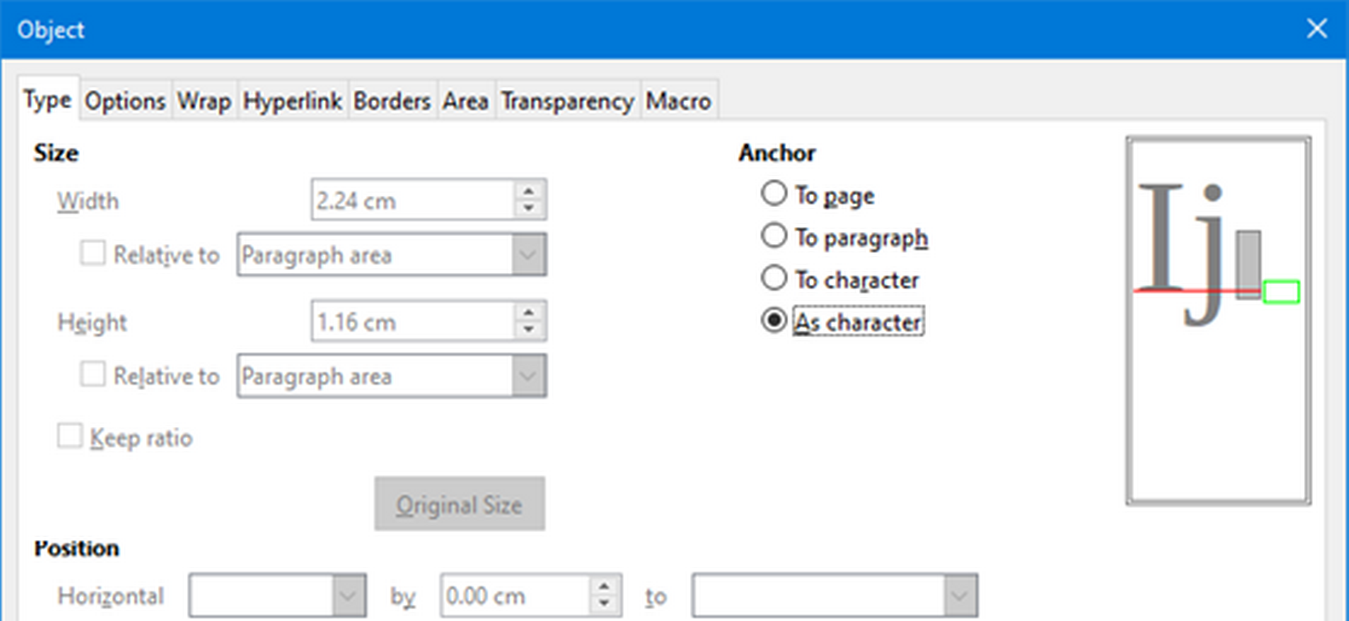Note

The anchoring options are not available in the Object dialog when making changes to the options available for frame styles. For more information on how to modify frame styles, please refer to the chapters on styles in the Writer Guide.

## Vertical alignment

The normal default setting for vertical alignment for formula objects is to use the text base line as a reference. This default setting can be changed by modifying the Formula frame style; see the chapters on styles in the Writer Guide for more information.

To change the vertical alignment position of an individual formula object (assuming that the As character anchoring option is selected):

1)  Right-click on the selected formula object and select Properties in the context menu, or go to Format > Frame and Object > Properties to open the Object dialog (Figure 13).

2)  Make sure the Type tab is selected and select a new alignment position in the drop-down list in the Position section. The vertical alignment options available are Top, Bottom, Center, or From bottom.

3)  If necessary, type in the text box a plus or minus value for vertical alignment. This option is only available if From bottom vertical alignment has been selected.

4)  Select the type of text alignment in the drop-down list in the Position section. The text alignment options available are Base line, Character, and Row.

5)  Click OK to save changes and close the Object dialog.

Note

If the Position section in the Object dialog is grayed out and not available, then go to Tools > Options > LibreOffice Writer > Formatting Aids and uncheck the option Math baseline alignment. This setting is stored with the document and applies to all formulas within it. Any new documents created will also use this setting for Math baseline alignment.

## Object spacing

A formula object, when inserted into a Writer document, has spacing each side of the formula object. The default value used for spacing is set within the frame style for formula objects and can be changed by modifying the Formula frame style. See the chapters on styles in the Writer Guide for more information.

To individually adjust the spacing for each formula object within a document:

1)  Create a formula in a Writer document.

2)  Right-click on the selected formula object and select Properties in the context menu, or go to Format > Frame and Object > Properties to open the Object dialog.

3)  Click on the Wrap tab to open the Wrap page in the Object dialog (Figure 14).

4)  In the Spacing section, enter the spacing value for Left, Right, Top, and Bottom spacing.

5)  Click OK to save the changes and close the Object dialog.

Figure 14: Object dialog – Wrap tab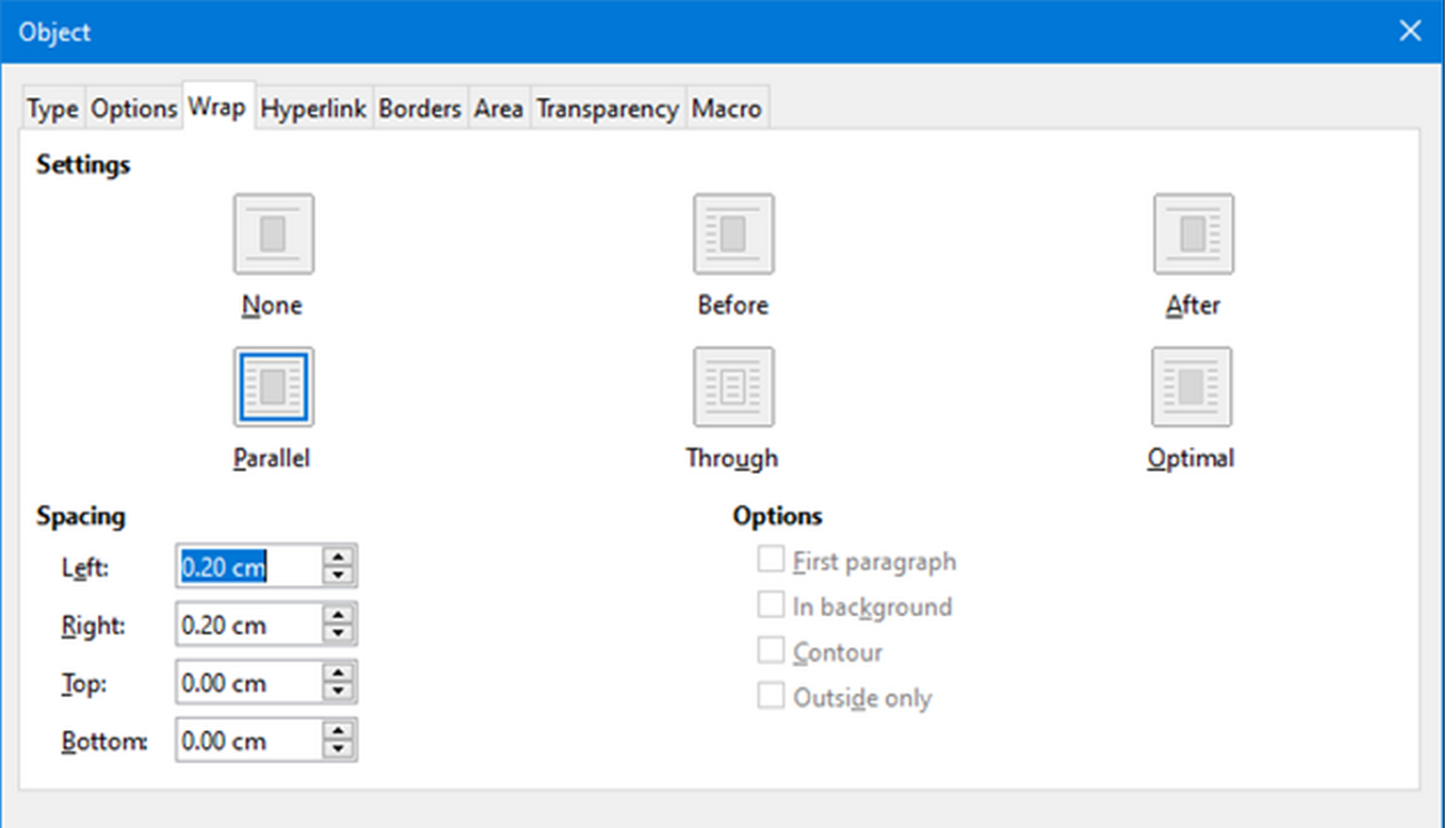## Text mode

In large formulas placed within a line of text, the formula elements can often be higher than the text height. Therefore, to make large formulas easier to read, it is recommended to always insert large formulas into a separate paragraph of their own so that it is separated from text.

However, if it is necessary to place a large formula within a line of text, double-click on the formula to open the Formula Editor and then go to Format > Text Mode on the Menu bar. The Formula Editor will try to shrink the formula to fit the text height. The numerators and denominators of fractions are shrunk, and the limits of integrals and sums are placed beside the integral/sum sign, as shown in the following example.

Example

A formula in a separate paragraph:

$∑i=25i2$

and the same formula embedded into a line of text using text mode format: $\sum _{i=2}^{5}{i}^{2}$

## Background and borders

The default setting for background (area fill) and borders for formula objects is set by the Formula frame style. To change the default setting for the Formula frame style, refer to the chapters on styles in the Writer Guide. However, for individual formulas in a document, the background and borders can be changed.

Note

The size of the frame that a formula is placed in when inserted into a document cannot be changed. The frame size for a formula object depends on the setting of the formula font size. See “Formula font size” on page 1 for more information.

Backgrounds

1)  In the document, select the formula for the background change.

2)  Right-click on the formula and select Properties in the context menu, or go to Format > Frame and Object > Properties to open the Object dialog.

3)  Click on the Area tab and use the buttons at the top to select the desired type of fill for the formula (Figure 15).

4)  Select the options for the formula background. The options change depending on the type of fill selected.

5)  Click OK to save changes and close the Object dialog.

Figure 15: Object dialog – Area tab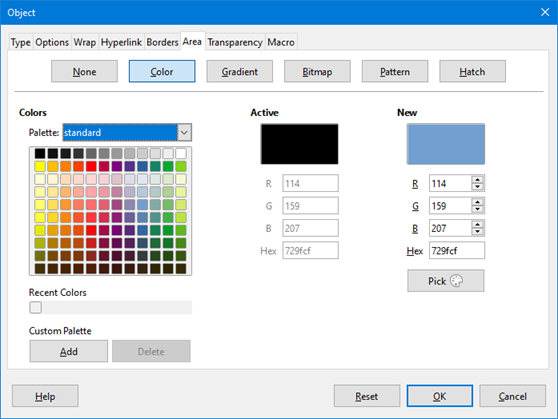Borders

1)  In the document, select the formula for the border change.

2)  Right-click on the formula and select Properties in the context menu, or go to Format > Frame and Object > Properties to open the Object dialog.

3)  Click on the Borders tab and select the options for the formula borders (Figure 16).

4)  Click OK to save changes and close the Object dialog.

Figure 16: Object dialog – Borders tab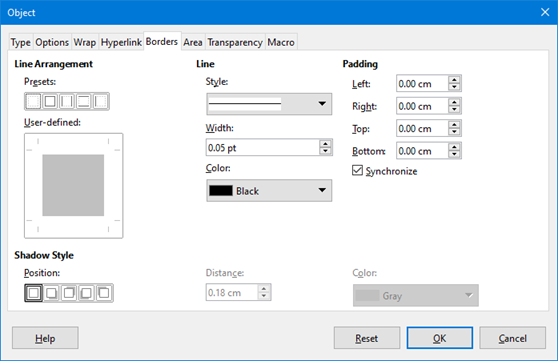## Quick insertion of formulas

To quickly insert formula markup language into a Writer document without opening the Formula Editor:

1)  Enter the formula markup language into document at the position where it should be placed.

2)  Select the markup language.

3)  Go to Insert > Object on the Menu bar and select Formula Object to create a formula from the selected markup language.

4)  Alternatively use the key combination Ctrl + Insert to open the Insert OLE Object dialog and then select Formula.

# Formulas in Calc, Draw, and Impress

In Calc, Draw, and Impress, formulas are inserted as OLE objects without any background (area fill) or borders. Each formula object is inserted into a spreadsheet, drawing, or slide as follows:

• In Calc, formulas are inserted into a selected cell in a spreadsheet with no style assigned to the formula object.

• In Draw and Impress, formulas are inserted into a central position on a drawing or slide and, by default, are assigned the drawing object style Object with no fill and no line. For more information on how to modify or assign drawing object styles, see the Draw Guide or the Impress Guide.

## Anchoring formulas

### Calc

A formula object can be anchored into a spreadsheet as To Page (default setting), or as To Cell. To change the anchoring type of formulas in a Calc spreadsheet:

1)  Select the formula object in the spreadsheet.

2)  Right-click on the formula and select Anchor > To Page or To Cell in the context menu.

3)  Alternatively, go to Format > Anchor on the Menu bar and select To Page or To Cell.

Tip

If an inserted formula in a Calc spreadsheet appears out of scale, fix it by right-clicking the formula object and then selecting the Original Size option in the context menu.

### Draw and Impress

When a formula is inserted into a drawing or slide, it is inserted as a floating OLE object and is not anchored to any particular position in the drawing or slide.

## Formula object properties

Formula objects in Calc, Draw, and Impress can be modified just like any other object that has been placed in a spreadsheet, drawing, or presentation, with the exception of formula object size and changing the format of any text within a formula. For more information on how to change object properties, see the Calc Guide, Draw Guide and Impress Guide.

The following points will help determine which dialog to use to change the properties of formula objects.

• For formula backgrounds, use the various options in the tabs of the Area dialog.

• For formula borders, use the various options in the Line dialog. Note that formula borders are separate from cell borders in a Calc spreadsheet.

• To accurately re-position a formula object, use the various options in tabs of the Position and Size dialog.

• In Draw and Impress, formula objects can be arranged, aligned, grouped, flipped, converted, combined, and edited.

• The text attributes of a formula object cannot be changed. The text used in a formula is set when creating the formula in the Formula Editor.

• Formula object size is set by the formula font size when the formula is created in the Formula Editor. The formula object size is protected in the Position and Size dialog, but this can be deselected if desired. However, this is not recommended as resizing a formula object using the Position and Size dialog could lead to distortion of the formula making it difficult to read.

## Formulas in charts

A chart in a Calc spreadsheet is itself an OLE object, therefore, the Formula Editor cannot be used to create and insert a formula directly into a chart. However, both the Chart and Math objects can be created separately and late copy and paste the Math formula into the Chart object:

1)  Create the chart using LibreOffice Calc. For a complete reference on how to create charts, see Chapter 3 in the Calc Guide.

2)  Click at any cell in the spreadsheet so that the Chart is no longer selected.

3)  Insert a Math Formula object by clicking Insert > Object > Formula.

4)  Type the desired formula into the Formula Editor.

5)  After editing the formula, select the Math Formula object and press Ctrl+C to copy the Formula object to the clipboard.

6)  Double-click the chart object to start editing the chart and press Ctrl+V to paste the Formula object into the chart.

7)  Now the object can be positioned anywhere inside the chart.

To change the formula, repeat the whole process of creating, copying, and pasting the Formula object into the chart.

## Chemical formulas

The primary purpose of Math is to create mathematical formulas, but it can also be used to write chemical formulas. However, in chemical formulas, the chemical symbols are normally written in uppercase using non-italic characters.

To create chemical formulas using Math, change the font used for variables to a non-italic font. For more information on how to change fonts in a formula, see “Formula fonts” on page 1.

For reversible reactions in chemical formulas, there is no symbol for a double arrow in Math. With access to a font with correct symbols for use in chemical formulas, these symbols can be added to the Catalog. See “Catalog customization” on page 1 for more information.

# Customization

This section explains how to customize LibreOffice Math formula creation in LibreOffice documents. Also, refer to Chapter 13, Customizing LibreOffice, for more general information on how to customize LibreOffice.

Keyboard shortcuts can be added to LibreOffice to make creating documents much easier. See Chapter 13, Customizing LibreOffice, for instructions.

## Catalog customization

For regular use of a symbol that is not available in Math, add it to the Symbols dialog (Figure 17) by using the Edit Symbols dialog (Figure 18).

Symbols can be added to a symbol set, symbol sets edited, symbol notations modified, new symbol sets defined, names assigned to symbols, existing symbol sets modified.

For details of the fields in the Edit Symbols dialog, please refer to the Math Guide.

1)  Go to Tools > Symbols on the Menu bar or click on the Symbols icon on the Tools toolbar to open the Symbols dialog.

2)  Click the Edit button to open the Edit Symbols dialog.

3)  Select a font in the Font drop-down list.

4)  Scroll down and select a symbol character to be added in the preview box. The small right preview box displays the new symbol.

5)  In the Symbol box, name the symbol being added.

6)  In the Symbol set box, select a symbol set in the drop-down list to add the new symbol to, or type a new name to create a new symbol set for the new symbol.

7)  If required, select a font style in the Style drop-down list – Standard, Italic, Bold, or Bold, Italic.

8)  Click Add, then click OK to close the Edit Symbols dialog. The new symbol and, if created, new symbol set are now available for use.

Figure 17: Symbols dialog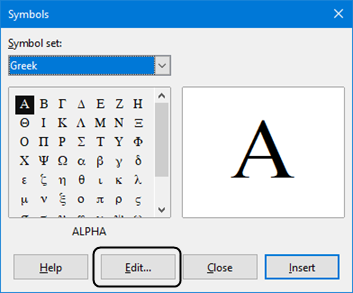Figure 18: Edit Symbols dialog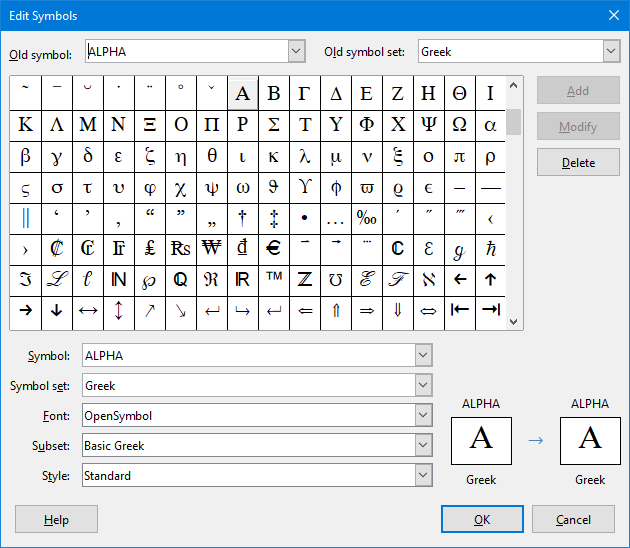Note

After a new symbol is added to the catalog, it can be added to a new formula by typing a percentage sign (%) followed by the new name into the markup language. Remember that symbol names are case sensitive, for example, %prime is a different symbol to %Prime.

Note

By default, only those user-defined symbols that actually occur in the document are stored with it. Sometimes it is useful to embed all the user-defined symbols, so that when the document is transferred to another computer it can be edited by another person. Go to Tools > Options > LibreOffice Math > Settings on the Menu bar and uncheck Embed only used symbols (smaller file size) in the Miscellaneous Options section.

## Editing symbols

### Modifying symbol names

To change the name of a symbol:

1)  In the Edit Symbols dialog (Figure 18), select the symbol name to be changed in the Old symbol drop-down list. The symbol appears in the left preview pane at the bottom of the Edit Symbols dialog (Figure 18).

2)  Type a new name for the symbol in the Symbol text box, or select a new name in the Symbol drop-down list. The new symbol name appears above the right preview pane at the bottom of the Edit Symbols dialog.

3)  Click Modify and the symbol name is changed.

4)  Click OK to close the Edit Symbols dialog.

### Moving symbols

To move a symbol from one symbol set to another:

1)  In the Edit Symbols dialog (Figure 18), in the Old symbol set drop-down list, select the symbol set where the symbol to be moved is located.

2)  Select the symbol name to be moved in the Old symbol drop-down list. The symbol appears in the left preview pane at the bottom of the Edit Symbols dialog (Figure 18).

3)  In the Symbol set drop-down list, select the desired symbol set to move the symbol to. The new symbol set name appears below the right preview pane at the bottom of the Edit Symbols dialog.

4)  Click Modify and the symbol is moved to the new symbol set.

5)  Click OK to close the Edit Symbols dialog.

### Deleting symbols

To delete a symbol from a symbol set:

1)  In the Edit Symbols dialog (Figure 18), in the Old symbol set drop-down list, select the symbol set from which the symbol should be deleted.

2)  Select the symbol name to be deleted in the Old symbol drop-down list. The symbol appears in the left preview pane at the bottom of the Edit Symbols dialog (Figure 18).

3)  Click Delete and the symbol is deleted from the symbol set without any confirmation.

4)  Click OK to close the Edit Symbols dialog.

Note

The only way to delete a symbol set is by deleting all of the symbols in that set. When the last symbol is deleted from a set, the set is also deleted.

## Formula spacing

The grave accent () inserts an additional small space and the tilde (~) inserts an additional large space into formulas. However, in the basic installation of LibreOffice, these symbols are ignored when they occur at the end of a formula. For running text in a formula, it may be necessary to include spacing at the end of formulas as well. This customization is only required when working with a Math document and is not required when inserting a formula into another LibreOffice component.

To add spacing at the end of formula in Math, go to Tools > Options > LibreOffice Math > Settings on the Menu bar and uncheck Ignore ~ and ` at the end of the line in the Miscellaneous Options section.

Note

To customize LibreOffice Math settings, open LibreOffice Math and then navigate to Tools > Options > LibreOffice Math > Settings.

## Scaling of code in the Formula Editor input window

By default, the code in the Formula Editor input window (Figure 7) is scaled to 100%. The scaling factor can be changed by going to Tools > Options > LibreOffice Math > Settings on the Menu bar and changing the percent in the Scaling code input window box in the Miscellaneous Options section. For example, if the scaling is changed to 150%, the code in the input window will appear larger. This setting affects the Formula Editor in all LibreOffice components. However, the LibreOffice Math section in the Options dialog can only be accessed from within the Math component. It is not available when editing a formula in a document in any other LibreOffice component.

## Extensions

For frequent formula creation and editing, LibreOffice can be customized by adding extensions that are designed to help create formulas. Extensions are easily installed using the Extension Manager. For more information on how to install extensions, see Chapter 13, Customizing LibreOffice.

A commonly used extension is Formatting of All Math Formulas. It allows the formatting of all Math formulas in a Writer, Calc, Draw, or Impress document. With it the font names and font sizes of all formulas can be changed in a document. For more information on this extension, go to https://extensions.libreoffice.org/en/extensions/show/formatting-of-all-math-formulas.

# Exporting and Importing

## Math ML format

In addition to exporting documents as PDFs, LibreOffice offers the possibility of saving formulas in the MathML format. This allows the insertion of formulas into documents that were created in other software, for example, Microsoft Office or an internet browser.

Note

Some internet browsers do not fully support the MathML format, so formulas may not display correctly.

If working on a Math document, go to File > Save as on the Menu bar or use the keyboard combination Ctrl+Shift+S to open the Save as dialog. Select MathML in the list of available file formats in File type to save the formula as MathML.

If working in another LibreOffice component, right-click on the formula object and select Save Copy as in the context menu to open the Save as dialog. Select MathML in the list of available file formats in File type to save the formula object as MathML.

MathML formulas can be imported into Math by using Tools > Import MathML from Clipboard on the Menu bar.

## Microsoft file formats

To control how formulas in Microsoft format are imported and exported using LibreOffice, go to Tools > Options > Load/Save > Microsoft Office on the Menu bar and select or deselect the options for MathType to LibreOffice Math or reverse.

• [L]: Load and convert the object

• [S]: Convert and save the object

### [L]: Load and convert the object

Select this option if Microsoft OLE objects are to be converted into the specified LibreOffice OLE objects when a Microsoft document is opened in LibreOffice. For formulas, any embedded MathType objects must not exceed the MathType 3.1 specifications to be successfully loaded and converted. Information on MathType format can be found at https://docs.wiris.com/en/mathtype/office_tools/microsoft_office.

If a document containing OMML formulas has been saved in .docx format and then converted to the older .doc format, then any OMML objects are converted into graphics, which will be displayed in LibreOffice as graphics.

### [S]: Convert and save the object

Select this option if LibreOffice OLE objects are to be converted and saved in Microsoft file format. LibreOffice converts any formulas into a format that can be read and modified by Microsoft Equation Editor and MathType.

When this option is not selected, the formula is treated as an OLE object on conversion into a .doc format and remains linked to LibreOffice. A double-click on the object in Microsoft Office will attempt to launch LibreOffice.